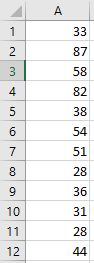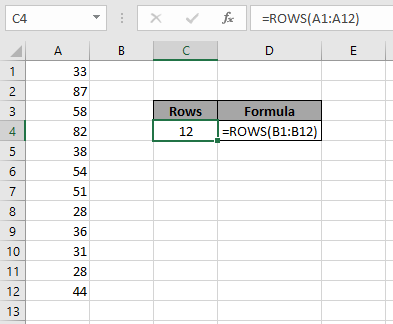# Total number of rows in range in excel

In this article, we will learn how to get the number of rows in a range of data using ROWS function in Excel.

The ROWS function returns the total number of rows when a range of cells is given as an argument
Syntax:

=ROWS(array or range)

Array or range: provided as argument in the function
Let’s learn this through an example shown below.
Here we have numbers in one column and we need to find the total number of rows in the list.Use the formula to get the number of rows in a array

=ROW(A1:A12)

Here Range A1:A12 is provided as argument in the functionAs you can see the formula returns the number of rows in an array.

Hope you learned how to get the total number of rows using ROWS function in Excel. Explore more articles here on Excel ROW & COLUMN functions. Please feel free to state your query or feedback in the comment section below. We will help you.

Related Articles:

Count table rows & columns in Excel

Excel Row function

How To Switch Rows and Columns in Excel

Popular Articles:

The VLOOKUP Function in Excel

COUNTIF in Excel 2016

How to Use SUMIF Function in Excel

Terms and Conditions of use

The applications/code on this site are distributed as is and without warranties or liability. In no event shall the owner of the copyrights, or the authors of the applications/code be liable for any loss of profit, any problems or any damage resulting from the use or evaluation of the applications/code.